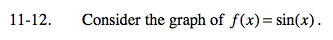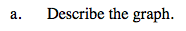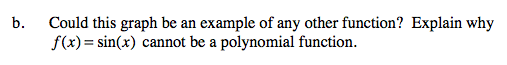Home > A2C > Chapter 11 > Lesson 11.1.1 > Problem11-12

11-12.
1. Consider the graph of f(x) = sin(x). Homework Help ✎

1. Describe the graph.

2. Could this graph be an example of any other function? Explain why f(x) = sin(x) cannot be a polynomial function.In general terms, what does it look like?How many roots does f(x) = sin(x) have?